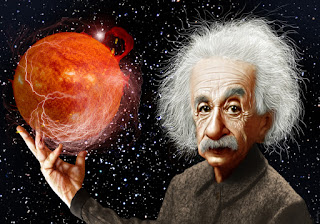Integration of Gaussian integral pops up in a lot of branches of physics and also a common homework problem. It cannot be evaluated by normal substitution method, you need to apply some tricks. There are several ways to solve it. Most common way is to combine two dimensional Gaussian. In this post I will explain how to do it. I don't just want to give you the answer. That's why after explaining few lines I have added "Show more" button. I want you guys to try it first, if you still can't then click "Show more" for more hints.Let \begin{align} I=\int_{-\infty}^{\infty}e^{-mx^2}dx \qquad \text{;where $m>0$}\label{eq:1} \end{align}
But hey, $x$ is just a dummy variable. If we change it to $y$ this integration will still be the same.
\begin{align} I=\int_{-\infty}^{\infty}e^{-my^2}dy \label{eq:2} \end{align} Just for fun, let's multiply $\eqref{eq:1}$ and $\eqref{eq:2}$ \begin{align} I^2=\int_{-\infty}^{\infty}e^{-mx^2}dx \int_{-\infty}^{\infty}e^{-my^2}dy \end{align} Using the rule of integration we can write it as \begin{align} I^2=\int_{-\infty}^{\infty} \int_{-\infty}^{\infty}e^{-m(x^2+y^2)}dx dy \end{align} This equation looks like something that could be evaluated in polar coordinates. That's what we are going to do next.... We need the integrating factor to Jacobian $r$. We know the regular old polar coordinate statement is $$r^2=x^2+y^2$$ So, let's go ahead and convert this integral into polar coordinates. The limit for $r$ will be $(0,\infty)$ but the limit for $\theta$ will be $(0,2\pi)$
So \begin{align} I^2=\int_{0}^{2\pi} \int_{0}^{\infty}re^{-mr^2}dr d\theta \end{align} Look, the term $re^{-mr^2}$ has no independence on $\theta$. So we get \begin{align} I^2=2\pi \int_{0}^{\infty}re^{-mr^2}dr \end{align} Hmm, looks better. May be we can try using substitution method this time..... Let $$u=r^2$$ \begin{align*} \dfrac{du}{dr}&=2r\\ \Rightarrow dr &=\dfrac{du}{2r} \end{align*} And the limit will still be $(0,\infty)$. So \begin{align*} I^2 &=2\pi \int_{0}^{\infty}re^{-mu} \dfrac{du}{2r}\\ &=\pi \int_{0}^{\infty}e^{-mu} du\\ \end{align*} Looks awesome. Now this integral is really easy to evaluate, isn't it? Let's evaluate it..... \begin{align*} I^2 &=\pi \dfrac{1}{m}(-e^{-mu}\bigg|_0^{\infty})\\ \Rightarrow I^2 &=\dfrac{\pi}{m}\\ \Rightarrow I &=\sqrt{\dfrac{\pi}{m}} \end{align*} And that's how you evaluate the Gaussian integral.Tricks to evaluate Gaussian IntegralReviewed by Dayeen on 3:25:00 AM Rating: 5